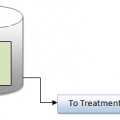## Calculation of Normality

Calculation of Normality

Equivalent weight (EW) = Molecular weight (MW) / Valency (Z)

Normality (N) =Equivalent weight (EW) / Volume of Solution (1000 ml.)

Meq = Actual weight (W) / Equivalent weight (EW)

Example: Molecular wt of K2Cr2O7 is 294.148 gm

Valency = 6 (because of Cr -6)

EW = 294.148/6 = 49.025

N = 49.025/1000 ml = 0.049025.

To make 0.25 N solution:

= 0.25 x EW = 0.25 x 49.025

= 12.256 gm. of K2Cr2O7 is required.

Molarity = Molecular Weight, gms / One Liter of Distilled Water

Example

1 M NaCl = 58.5 gm NaCl in 1 Ltr of D.W. = 58500 mg/Ltr

0.5 M NaCl = 29250 mg/Ltr toxic for Bacteria## Author:T.P.Sivanandan

#### Why Fine Bubbles are Better than Coarse bubble in Aeration Tank#### How to Calculate Lime Dosage Requirement in Sedimentation Process